# Composition closed premodel structures and the Kreweras lattice

no code yet • 7 Sep 2022

We investigate the rich combinatorial structure of premodel structures on finite lattices whose weak equivalences are closed under composition.

Algebraic Topology Category Theory 18N40, 06Bxx

TWEETS# The $P=W$ conjecture for $\mathrm{GL}_n$

no code yet • 6 Sep 2022

We prove the $P=W$ conjecture for $\mathrm{GL}_n$ for all ranks $n$ and curves of arbitrary genus $g\geq 2$.

Algebraic Geometry Representation Theory

TWEETS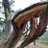# $P=W$ via $H_2$

no code yet • 12 Sep 2022

Let $H_2$ be the Lie algebra of polynomial Hamiltonian vector fields on the symplectic plane.

Algebraic Geometry Differential Geometry Representation Theory

TWEETS# Segal conditions for generalized operads

no code yet • 29 Aug 2022

This note is an introduction to several generalizations of the dendroidal sets of Moerdijk--Weiss.

Category Theory Algebraic Topology 18M85 (Primary) 18F20, 18M60, 55P48, 55U10, 05C20 (Secondary)

TWEETS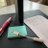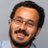# Stochastic Mathematical Systems

no code yet • 1 Sep 2022

To ground thinking, we understand the answers to questions given by this oracle to be the answers that would be given by an SMS representing the entire mathematical community in the infinite long run of the process of asking and answering questions.

Logic History and Philosophy of Physics

TWEETS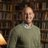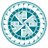# A new Lattice Boltzmann scheme for linear elastic solids: periodic problems

no code yet • 31 Aug 2022

We propose a new second-order accurate lattice Boltzmann scheme that solves the quasi-static equations of linear elasticity in two dimensions.

Numerical Analysis Numerical Analysis Applied Physics

TWEETS# Solving Fredholm Integral Equations of the First Kind via Wasserstein Gradient Flows

16 Sep 2022

Solving Fredholm equations of the first kind is crucial in many areas of the applied sciences.

Optimization and Control Numerical Analysis Functional Analysis Numerical Analysis Computation Methodology 65Rxx, 65C35, 65K10, 45B05

TWEETS# On the complexity of finding stationary points of smooth functions in one dimension

no code yet • 15 Sep 2022

We characterize the query complexity of finding stationary points of one-dimensional non-convex but smooth functions.

Optimization and Control

TWEETS# Applications of the algebraic geometry of the Putman-Wieland conjecture

no code yet • 1 Sep 2022

We give three applications of our prior work toward the Putman-Wieland conjecture.

Algebraic Geometry Geometric Topology 14H10, 14H45, 57K20

TWEETS# An introduction to the algebraic geometry of the Putman-Wieland conjecture

no code yet • 1 Sep 2022

We give algebraic and geometric perspectives on our prior results toward the Putman-Wieland conjecture.

Algebraic Geometry Geometric Topology 14D05, 14H10, 57K20

TWEETS